# Optimal Transport in 1-D¶

Important: Please read the installation page for details about how to install the toolboxes. $\newcommand{\dotp}{\langle #1, #2 \rangle}$ $\newcommand{\enscond}{\lbrace #1, #2 \rbrace}$ $\newcommand{\pd}{ \frac{ \partial #1}{\partial #2} }$ $\newcommand{\umin}{\underset{#1}{\min}\;}$ $\newcommand{\umax}{\underset{#1}{\max}\;}$ $\newcommand{\umin}{\underset{#1}{\min}\;}$ $\newcommand{\uargmin}{\underset{#1}{argmin}\;}$ $\newcommand{\norm}{\|#1\|}$ $\newcommand{\abs}{\left|#1\right|}$ $\newcommand{\choice}{ \left\{ \begin{array}{l} #1 \end{array} \right. }$ $\newcommand{\pa}{\left(#1\right)}$ $\newcommand{\diag}{{diag}\left( #1 \right)}$ $\newcommand{\qandq}{\quad\text{and}\quad}$ $\newcommand{\qwhereq}{\quad\text{where}\quad}$ $\newcommand{\qifq}{ \quad \text{if} \quad }$ $\newcommand{\qarrq}{ \quad \Longrightarrow \quad }$ $\newcommand{\ZZ}{\mathbb{Z}}$ $\newcommand{\CC}{\mathbb{C}}$ $\newcommand{\RR}{\mathbb{R}}$ $\newcommand{\EE}{\mathbb{E}}$ $\newcommand{\Zz}{\mathcal{Z}}$ $\newcommand{\Ww}{\mathcal{W}}$ $\newcommand{\Vv}{\mathcal{V}}$ $\newcommand{\Nn}{\mathcal{N}}$ $\newcommand{\NN}{\mathcal{N}}$ $\newcommand{\Hh}{\mathcal{H}}$ $\newcommand{\Bb}{\mathcal{B}}$ $\newcommand{\Ee}{\mathcal{E}}$ $\newcommand{\Cc}{\mathcal{C}}$ $\newcommand{\Gg}{\mathcal{G}}$ $\newcommand{\Ss}{\mathcal{S}}$ $\newcommand{\Pp}{\mathcal{P}}$ $\newcommand{\Ff}{\mathcal{F}}$ $\newcommand{\Xx}{\mathcal{X}}$ $\newcommand{\Mm}{\mathcal{M}}$ $\newcommand{\Ii}{\mathcal{I}}$ $\newcommand{\Dd}{\mathcal{D}}$ $\newcommand{\Ll}{\mathcal{L}}$ $\newcommand{\Tt}{\mathcal{T}}$ $\newcommand{\si}{\sigma}$ $\newcommand{\al}{\alpha}$ $\newcommand{\la}{\lambda}$ $\newcommand{\ga}{\gamma}$ $\newcommand{\Ga}{\Gamma}$ $\newcommand{\La}{\Lambda}$ $\newcommand{\si}{\sigma}$ $\newcommand{\Si}{\Sigma}$ $\newcommand{\be}{\beta}$ $\newcommand{\de}{\delta}$ $\newcommand{\De}{\Delta}$ $\newcommand{\phi}{\varphi}$ $\newcommand{\th}{\theta}$ $\newcommand{\om}{\omega}$ $\newcommand{\Om}{\Omega}$

This tour details the computation of discrete 1-D optimal transport with application to grayscale image histogram manipulations.

In :
addpath('toolbox_signal')


## Optimal Transport and Assignement¶

We consider data $f \in \RR^{N \times d}$, that can corresponds for instance to an image of $N$ pixels, with $d=1$ for grayscale image and $d=3$ for color image. We denote $f = (f_i)_{i=1}^N$ with $f_i \in \RR^d$ the elements of the data.

The discrete (empirical) distribution in $\RR^d$ associated to this data $f$ is the sum of Diracs $$\mu_f = \frac{1}{N} \sum_{i=1}^N \de_{f_i}.$$

An optimal assignement between two such vectors $f,g \in \RR^{N \times d}$ is a permutation $\si \in \Si_N$ that minimizes $$\si^\star \in \uargmin{\si \in \Si_N} \sum_{i=1}^N C(f_i,g_{\si(i)})$$ where $C(u,v) \in \RR$ is some cost function.

In the following, we consider $L^p$ costs $$\forall (u,v) \in \RR^d \times \RR^d, \quad C(u,v) = \norm{u-v}^p$$ where $\norm{\cdot}$ is the Euclidean norm and $p\geq 1$.

This optimal assignement defines the $L^p$ Wasserstein distance between the associated point clouds distributions $$W_p(\mu_f,\mu_g)^p = \sum_{i=1}^N \norm{f_i - g_{\si(i)}}^p = \norm{f - g \circ \si}_p^p$$ where $g \circ \si = (g_{\si(i)})_i$ is the re-ordered points cloud.

## Grayscale Image Distribution¶

We consider here the case $d=1$, in which case one can compute easily the optimal assignement $\si^\star$.

Load an image $f \in \RR^N$ of $N=n \times n$ pixels.

In :
n = 256;
f = rescale( load_image('lena', n) );


Display it.

In :
clf;
imageplot(f);A convenient way to visualize the distribution $\mu_f$ is by computing an histogram $h \in \RR^Q$ composed using $Q$ bins $[u_k,u_{k+1})$. The histogram is computed as $$\forall k=1,\ldots,Q, \quad h(p) = \abs{\enscond{i}{ f_i \in [u_k,u_{k+1}) }}.$$

Number of bins.

In :
Q = 50;


Compute the histogram.

In :
[h,t] = hist(f(:), Q);


Display this normalized histogram. To make this curve an approximation of a continuous distribution, we normalize $h$ by $Q/N$.

In :
clf;
bar(t,h*Q/n^2); axis('tight');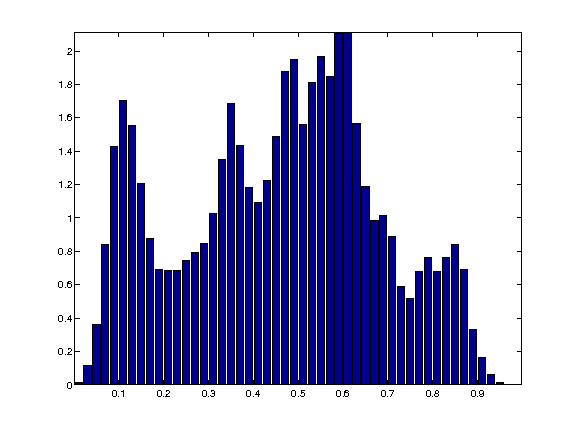Exercise 1

Compute and display the histogram of $f$ for an increasing number of bins.

In :
exo1()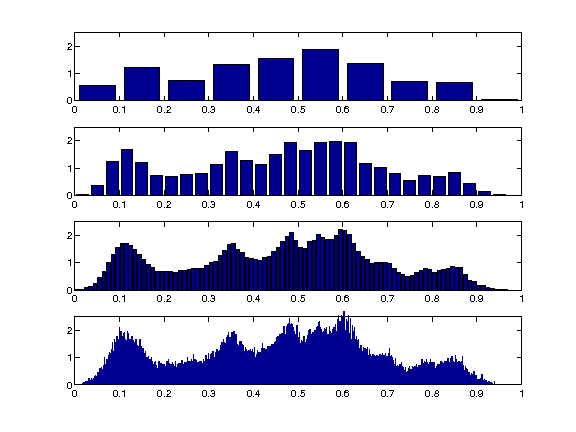In :
%% Insert your code here.


Load another image $g \in \RR^N$.

In :
g = rescale( mean(load_image('fingerprint', n),3) );


Display it.

In :
clf;
imageplot(g);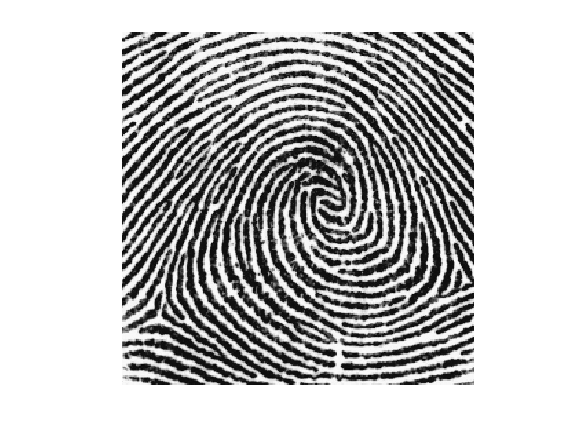Exercise 2

Compare the two histograms.

In :
exo2()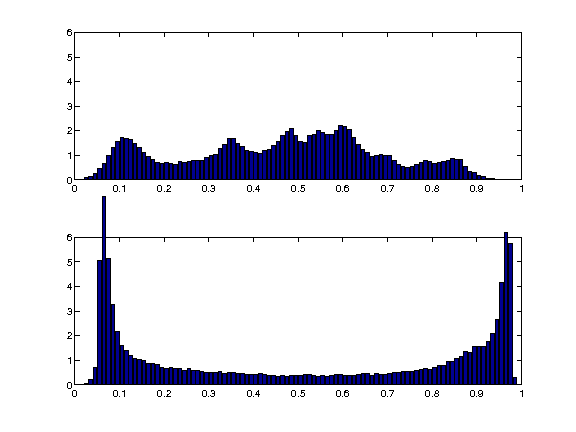In :
%% Insert your code here.


## 1-D Optimal Assignement¶

For 1-D data, $d=1$, one can compute explicitely an optimal assignement $\si^\star \in \Si_N$ for any cost $C(u,v) = \phi(\abs{u-v})$ where $\phi : \RR \rightarrow \RR$ is a convex function. This is thus the case for the $L^p$ optimal transport.

This is obtained by computing two permutations $\si_f, \si_g \in \Si_N$ that order the values of the data $$f_{\si_f(1)} \leq f_{\si_f(2)} \leq \ldots f_{\si_f(N)}$$ $$g_{\si_g(1)} \leq g_{\si_g(2)} \leq \ldots g_{\si_g(N)}.$$

An optimal assignement is then optained by assigning, for each $k$, the index $i = \si_f(k)$ to the index $\si_g(k)$, i.e. $$\si^\star = \si_g \circ \si_f^{-1}$$ where $\si_f^{-1}$ is the inverse permutation, that satisfies $$\si_f^{-1} \circ \si_f = \text{Id}$$.

Note that this optimal assignement $\si^\star$ is not unique when there are two pixels in $f$ or $g$ having the same value.

Compute $\si_f, \si_g$ in $O(N \log(N))$ operations using a fast sorting algorithm (e.g. QuickSort).

In :
[~,sigmaf] = sort(f(:));
[~,sigmag] = sort(g(:));


Compute the inverse permutation $\sigma_f^{-1}$.

In :
sigmafi = [];
sigmafi(sigmaf) = 1:n^2;


Compute the optimal permutation $\sigma^\star$.

In :
sigma = sigmag(sigmafi);


The optimal assignement is used to compute the projection on the set of image having the pixel distribution $\mu_g$ $$\Hh_g = \enscond{m \in \RR^N}{ \mu_m = \mu_g }.$$ Indeed, for any $p > 1$, the $L^p$ projector on this set $$\pi_g( f ) = \uargmin{m \in \Hh_g} \norm{ f - m }_p$$ is simply obtained by re-ordering the pixels of $g$ using an optimal assignement $\si^\star \in \Si_N$ between $f$ and $g$, i.e. $$\pi_g( f ) = g \circ \si^\star.$$

This projection $\pi_g( f )$ is called the histogram equalization of $f$ using the histogram of $g$

Compute the projection.

In :
f1 = reshape(g(sigma), [n n]);
imageplot(f1)Compare before/after equalization.

In :
clf;
imageplot(f, 'f', 1,2,1);
imageplot(f1, '\pi_g(f)',  1,2,2);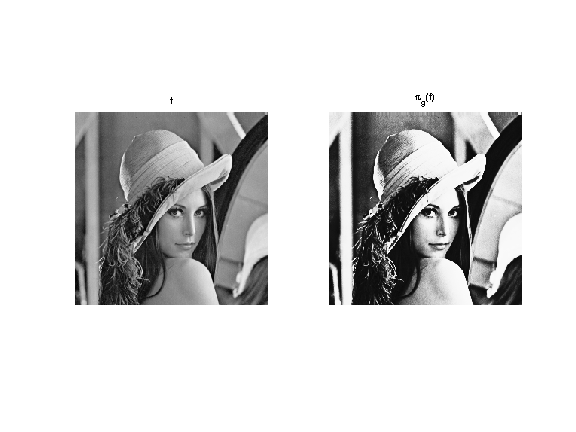## Histogram Interpolation¶

We now introduce the linearly interpolated image $$\forall t \in [0,1], \quad f_t = (1-t) f + t g \circ \sigma^{\star} .$$

One can show that the distribution $\mu_{f_t}$ is the geodesic interpolation in the $L^2$-Wasserstein space between the two distribution $\mu_f$ (obtained for $t=0$) and $\mu_g$ (obtained for $t=1$).

One can also show that it is the barycenter between the two distributions since it has the following variational characterization $$\mu_{f_t} = \uargmin{\mu} (1-t)W_2(\mu_f,\mu)^2 + t W_2(\mu_g,\mu)^2 .$$

Define the interpolation operator.

In :
ft = @(t)reshape( t*f1 + (1-t)*f, [n n]);


The midway equalization is obtained for $t=1/2$.

In :
clf;
imageplot(ft(1/2));Exercise 3

Display the progression of the interpolation of the histograms.

In :
exo3()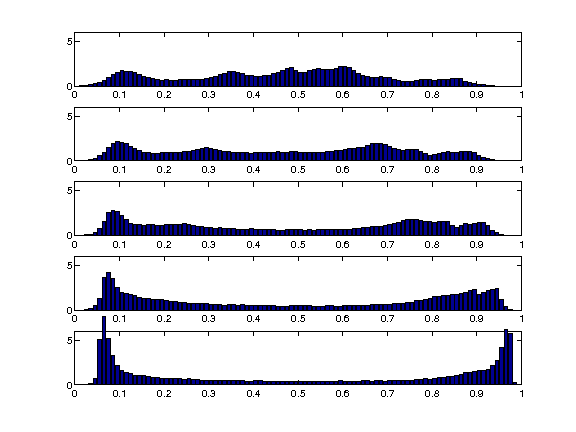In :
%% Insert your code here.

In :